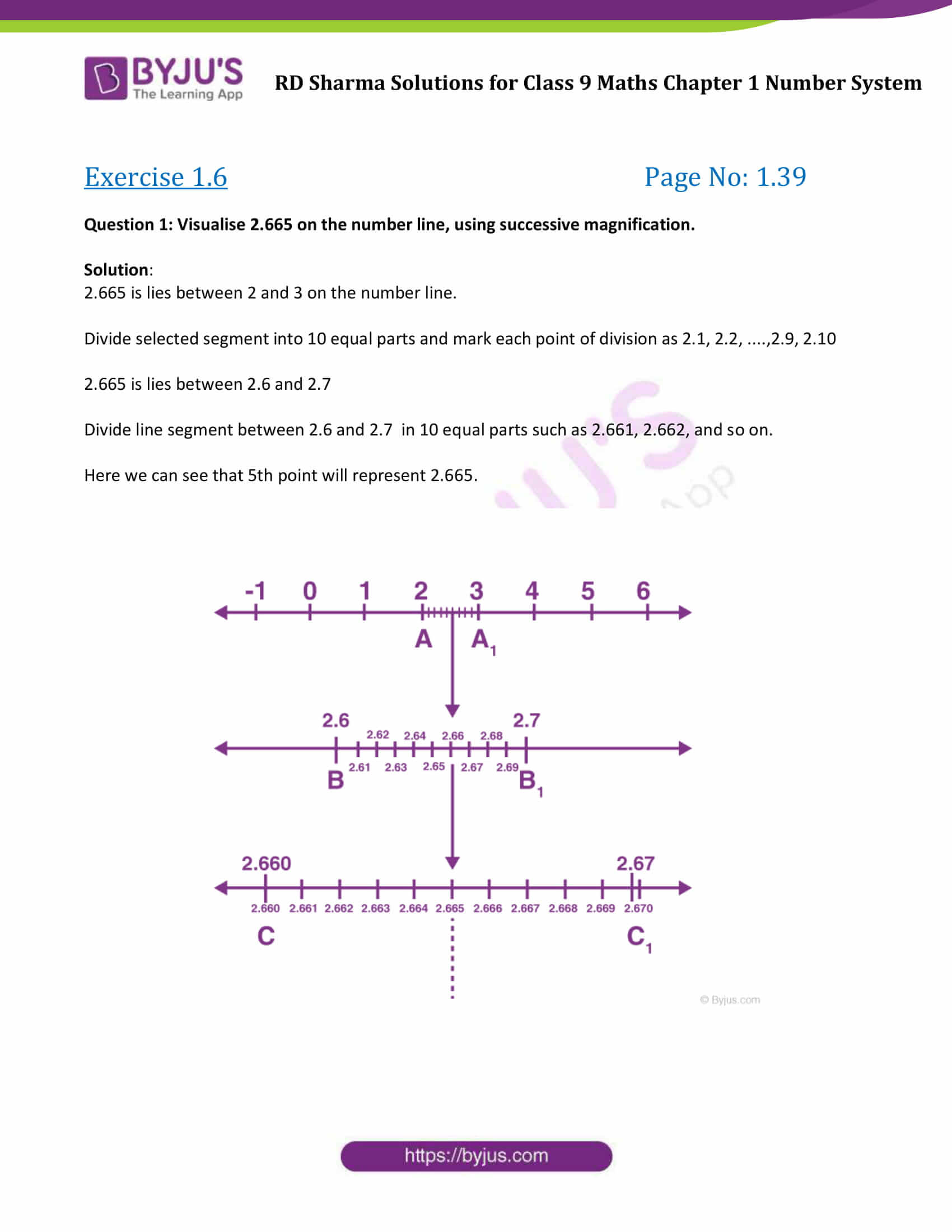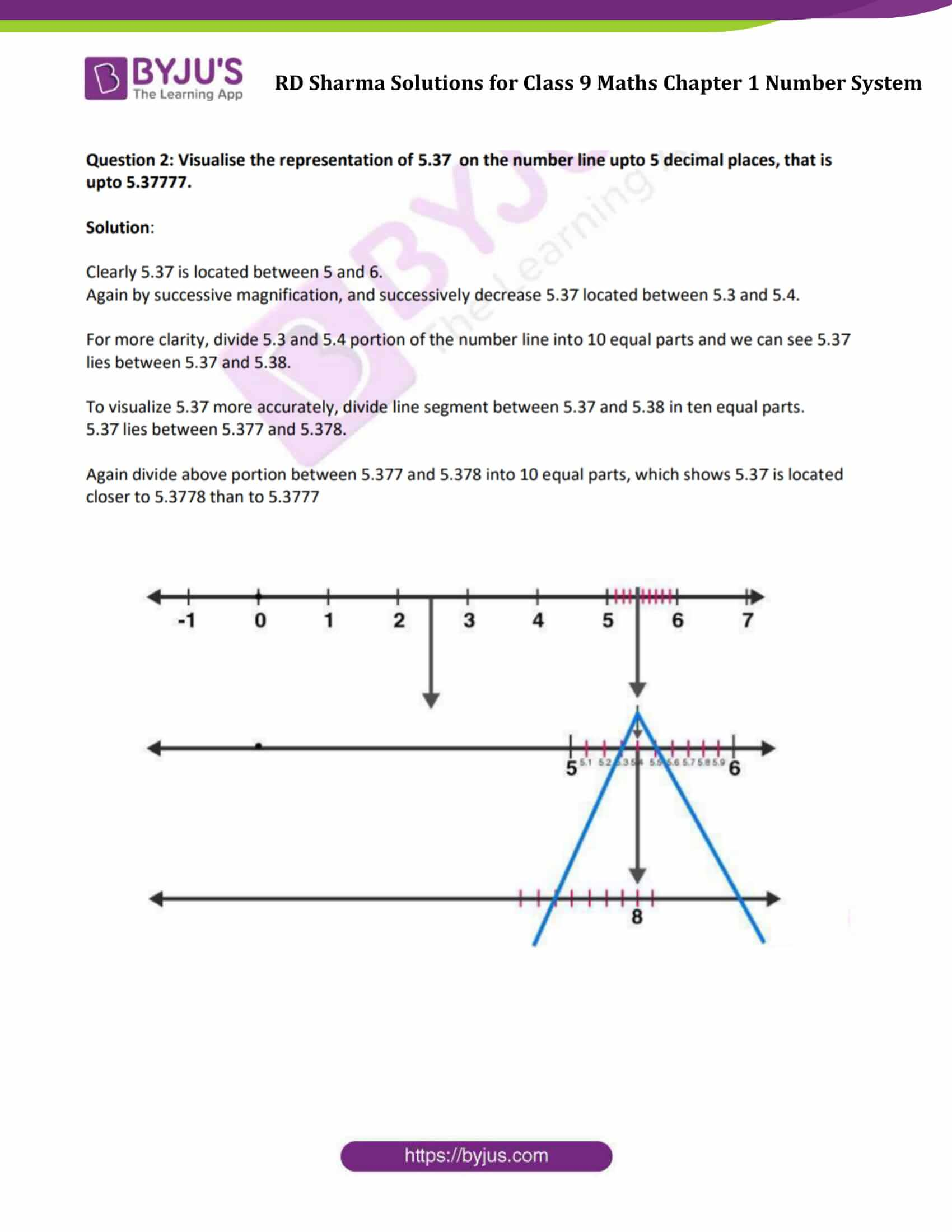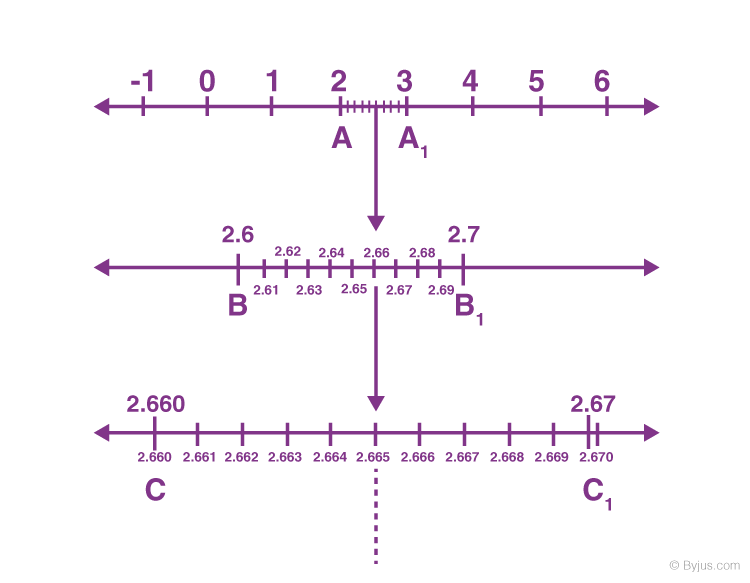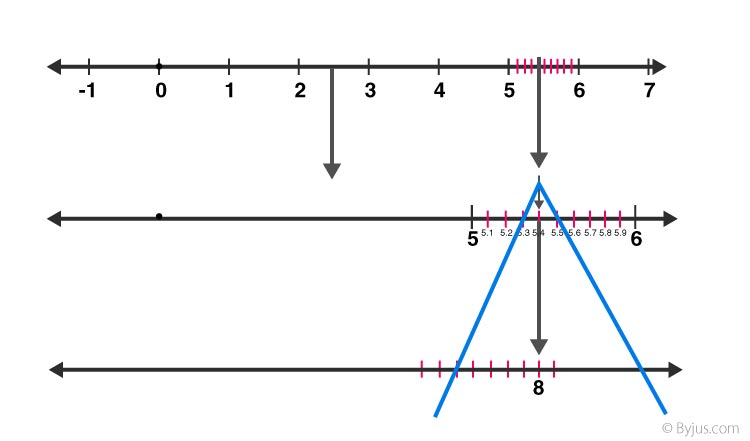# RD Sharma Solutions for Class 9 Maths Chapter 1 Number System Exercise 1.6

RD Sharma Class 9 Mathematics Chapter 1 Exercise 1.6 Number System is provided here. Exercise 1.6 is about visualisation of representation of real numbers by using the process of successive magnification. RD Sharma Solutions Class 9 chapter 1 are solved by experts in accordance with CBSE syllabus for 9th standard. This study material contains solved questions to the exercise 1.6 present in the RD Sharma class 9 textbook. Click on the link to download free PDF of chapter 1 exercise 1.6.

## Download PDF of RD Sharma Solutions for Class 9 Maths Chapter 1 Number System Exercise 1.6### Access Answers to Maths RD Sharma Solutions for Class 9 Chapter 1 Number System Exercise 1.6 Page number 1.39

Question 1: Visualise 2.665 on the number line, using successive magnification.

Solution:

2.665 is lies between 2 and 3 on the number line.

Divide selected segment into 10 equal parts and mark each point of division as 2.1, 2.2, ….,2.9, 2.10

2.665 is lies between 2.6 and 2.7

Divide line segment between 2.6 and 2.7 in 10 equal parts such as 2.661, 2.662, and so on.

Here we can see that 5th point will represent 2.665.Question 2: Visualise the representation of 5.37̅ on the number line upto 5 decimal places, that is upto 5.37777.

Solution:

Clearly 5.37̅ is located between 5 and 6.

Again by successive magnification, and successively decrease 5.37̅ located between 5.3 and 5.4.

For more clarity, divide 5.3 and 5.4 portion of the number line into 10 equal parts and we can see 5.37̅ lies between 5.37 and 5.38.

To visualize 5.37̅ more accurately, divide line segment between 5.37 and 5.38 in ten equal parts.

5.37̅ lies between 5.377 and 5.378.

Again divide above portion between 5.377 and 5.378 into 10 equal parts, which shows 5.37̅ is located closer to 5.3778 than to 5.3777